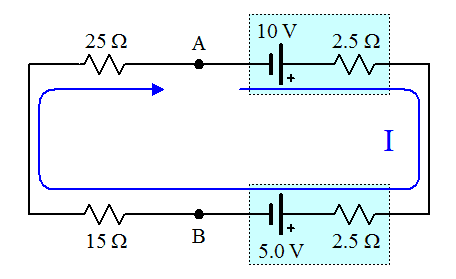# Simple question on current electricity

• Archived

## Homework Statement

Please refer to the diagram(attachment).....the circuit contains two resistors in series of 25 and 15 ohm respectively and there are two batteries of emf 10 V and 5 V respectively ....Only thing missing in the diagram is that both the batteries have internal resistances of 2.5 V.....We need the PD between pts A and B.....

## The Attempt at a Solution

Current in the circuit comes out to be 1/9....now applying Kirchoff's Law i am getting PD to be -(5-5/9) i.e -4.44 but answer is -4 the approximation is huge so i wanted to confirm my answer....

#### Attachments

• cir.bmp
238.1 KB · Views: 451

gneill
Mentor

With the two internal resistances for the batteries the situation appears as follows:The battery potentials are opposing, so there's 5 V of potential left to drive current. The current is thus:

##I = \frac{5~V}{(25 + 15 + 2×2.5)~Ω} = \frac{5}{45}~A = \frac{1}{9}~A##

The potential difference between A and B can be found by doing a "KVL walk" from B to A. Either direction around the loop would work, but choosing the left hand path through the external resistors the path is going with the current, so the potential drops over both resistors. The total drop is:

##V_{AB} = -I×R = -\frac{1}{9}~A×40~Ω = -\frac{40}{9}~V = -4.44~V##